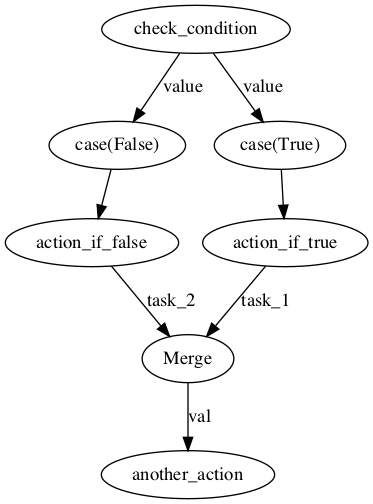Sometimes you have parts of a flow that you only want to run under certain conditions. To support this, Prefect provides several built-in tasks for control-flow that you can use to add conditional branches to your flow.

Let's say you want to have a flow where different tasks are run based on the result of some conditional task. In normal Python code this logic might look like:

``````if check_condition():
val = action_if_true()
else:
val = action_if_false()

another_action(val)
``````

To implement the same logic as a Flow, you can make use of Prefect's `case` blocks. Tasks added to a flow inside a `case` block are only run if the condition matches the case. Tasks in branches that aren't run will finish with a `Skipped` state.

We'll also need Prefect's `merge` function to merge the branches together before the `another_action` task. This function takes one or more tasks conditional tasks, and returns the output of the first task that isn't `Skipped`. This can be used to "merge" multiple branches in a flow together. If the results of `action_if_true`/`action_if_false` weren't needed later on in the flow, we wouldn't need this step.

The resulting flow looks like:Registering with Prefect Cloud/Server

This example can be registered in Prefect Cloud or Server by running:

``````prefect register --json https://docs.prefect.io/examples.json \
--project 'Prefect Examples'
``````

(to register in a different project, replace `'Prefect Examples'` with your project name).

``````from random import random

from prefect import task, Flow, case

def check_condition():
return random() < 0.5

def action_if_true():
return "I am true!"

def action_if_false():
return "I am false!"

def another_action(val):
print(val)

with Flow("Example: Conditional Tasks") as flow:
cond = check_condition()

with case(cond, True):
val_if_true = action_if_true()

with case(cond, False):
val_if_false = action_if_false()

val = merge(val_if_true, val_if_false)

another_action(val)

if __name__ == "__main__":
flow.run()
``````
Output
``````\$ python examples/conditional.py
INFO | Beginning Flow run for 'Example: Conditional Tasks'
INFO | SKIP signal raised: SKIP('Provided value "True" did not match "False"')